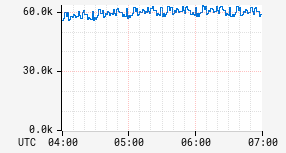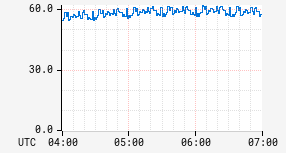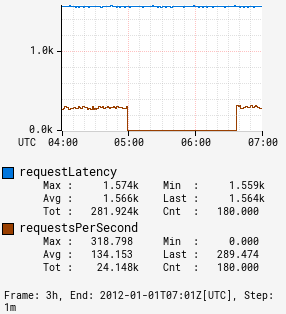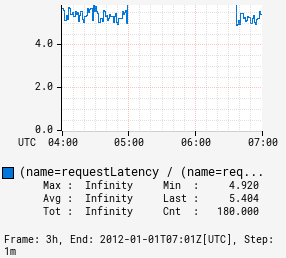# fdiv

Input Stack:
 ts2: TimeSeriesExpr ts1: TimeSeriesExpr
Output Stack:
 (ts1 / ts2): TimeSeriesExpr

Floating point division operator. Compute a new time series where each interval has the value `(a / b)` where `a` and `b` are the corresponding intervals in the input time series.

:fdiv 2.0 NaN Inf NaN NaN
Input 1 2.0 0.0 1.0 1.0 NaN
Input 2 1.0 0.0 0.0 NaN NaN

Note in many cases `NaN` will appear in data, e.g., if a node was brought up and started reporting in the middle of the time window for the graph. Zero divided by zero can also occur due to lack of activity in some windows. Unless you really need strict floating point behavior, use the div operator to get behavior more appropriate for graphs.

Example dividing a constant:

 Before After```name,sps,:eq ``` ```name,sps,:eq, 1024,:fdiv ```

Example dividing two series:

 Before After```name,requestLatency,:eq, :sum, name,requestsPerSecond,:eq, :max, (,name,),:by ``` ```name,requestLatency,:eq, :sum, name,requestsPerSecond,:eq, :max, (,name,),:by, :fdiv ```